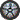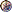### Prof. Paulo Franca - Spring 2000

Introduction

Syllabus

Guidelines for labs

Lab sections

Take quiz & check grades

## Searching in Graphs

### Breadth First SearchGiven a graph and a source vertex "s":Explore all edges to discover every vertex reachable from "s".Produce a tree "Breadth First Tree" that contains all such verticesComputes the distance (number of edges) to all verticesPath in the BF Tree is the shortest path to each vertex.Directed and undirected graphs

#### ApproachProgress uniformly in all directions (discovers all vertices at distance k before distance k+1)Use status or colors: white, gray , blackwhite - not discoveredgray - discovered but further edges are not exploredblack - discovered and explored

#### Build a tree starting at "s"s is the rootscan all adjacent vertices and add new edges to the treepredecessor or parent of vertex: u is parent of v if v was discovered from u

#### vertex information:info fieldparent vertexdistance in edgescolor

#### you need to represent a list of edges (adjacent nodes), suggestion: use an adjacency list.#### Algorithm approach:

build a Breadth First Tree from vertex "u" to vertex "v"

Initialize all vertices
Set up the source vertex;
color=gray, distance=0, parent=0;
put this node (s) in the queue Q.
Explore vertices in queue Q:
while (Q) not empty:
{
u = head of queue (Q); // remove it?
for each vertex "v", adjacent to u:
if  v->color = white
{
color =gray
distance=distance +1;
parent = u
put "v" in queue Q
}
Dequeue (Q)
u -> color = black
}

ta